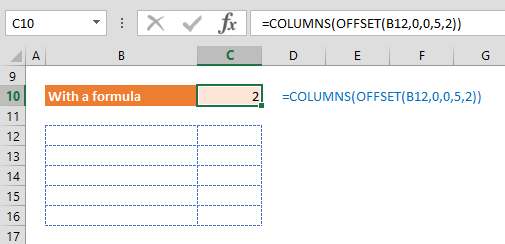The Excel COLUMNS function returns the number of columns in a selection. In this guide, we’re going to show you how to use this function and also go over some tips and error handling methods.

# Supported versions

• All Excel versions

# Syntax

SUMIF(range, criteria, [sum_range])

# Arguments

 array An array, an array formula, or a reference to a range of cells.

Examples

## Basic Scenario

=COLUMNS(B4:E8)
formula returns 4 as a count of the columns between columns B and E.## Using with a formula

You can use the COLUMNS function with an array or an array returning formula to make it dynamic. In our example, the OFFSET function returns an array of values in the range B12:C16, which is used as the array argument.

=COLUMNS(OFFSET(B12,0,0,5,2))## Generating number series

An alternative way of using the COLUMNS function is for generating number series. You can use an expanding reference as array argument. Expanding a range from \$C\$10:\$C10 to \$C\$10:\$C15 generates numbers from 1 to 6.

=COLUMNS(\$C\$18:C\$18)

.

.

.

=COLUMNS(\$C\$18:G\$18)# Tips

• Use the COLUMN function if you need the column number of a reference.
• To get the count of columns of an array or range, use the ROWS function.